An algebraic groupacting regularly on an algebraic variety. More precisely, it is a tripletwhere(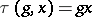) is a morphism of algebraic varieties satisfying the conditions: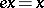,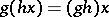for alland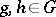(whereis the unit of). If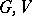andare defined over a field, then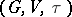is called an algebraic group of-transformations. For instance,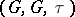, whereis the adjoint action or an action by shifts, is an algebraic group of transformations. Ifis an algebraic subgroup in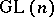andis its natural action on the affine space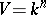, thenis an algebraic group of transformations. For each point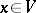one denotes bythe orbit of, and by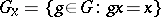the stabilizer of. The orbit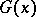need not necessarily be closed in, but closed orbits exist always, e.g. orbits of minimal dimension are closed. An algebraic group of transformations is sometimes understood to mean a groupwhich is acting rationally (but not necessarily regularly) on an algebraic variety(this means that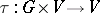is a rational mapping, and the above properties ofare valid for ordinary points). It was shown by A. Weil  that there always exists a variety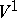, birationally isomorphic to, and such that the action ofoninduced by the rational action ofonis regular. The problem of describing the orbits, stabilizers, fields of invariant rational functions (cf. Invariants, theory of), and of constructing quotient varieties are fundamental in the theory of algebraic groups of transformations and have numerous applications.